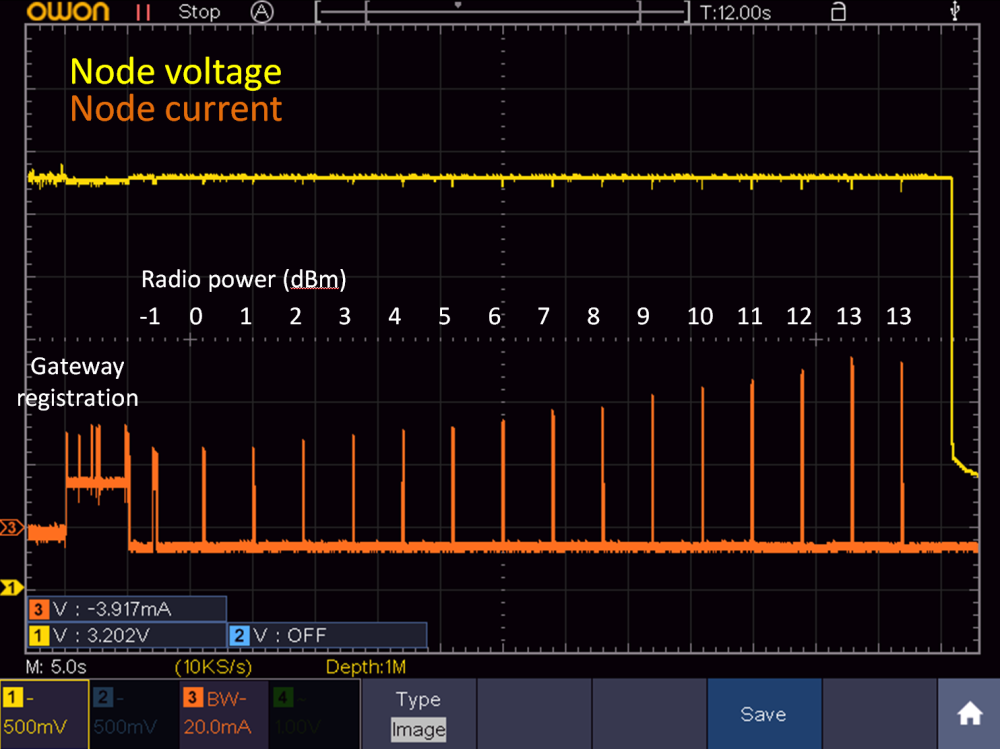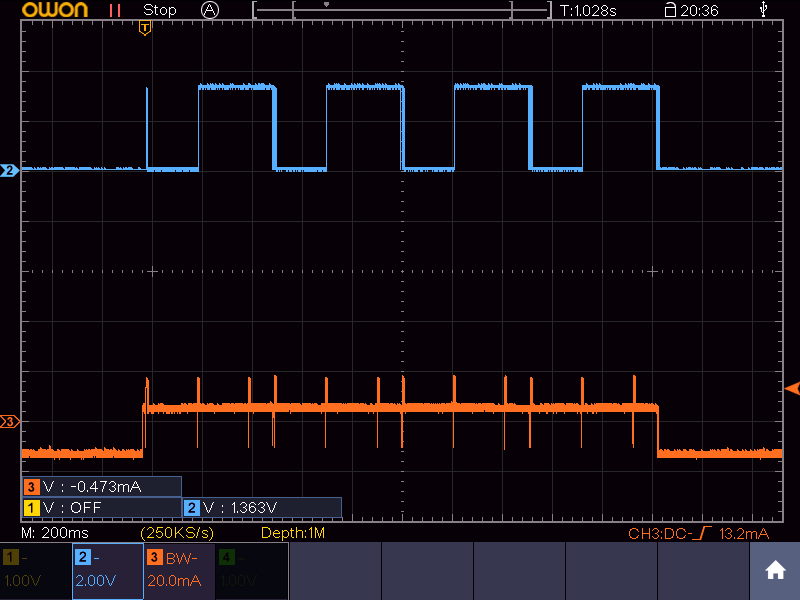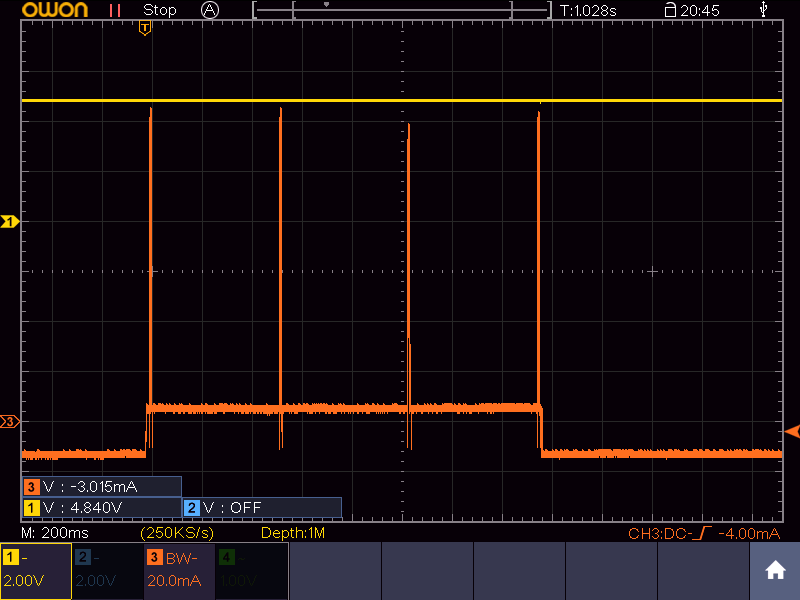# RFM69 ATC not working?

• I want to use my MySensors/RFM69HW node with the lowest power possible. However, with ATC enabled, I noticed that the power is always adjusted to the maximum level possible. To investigate, I did an experiment in which I disabled ATC and increased the radio power from -2dBm to 13dBm and have the node send the receiving RSSI and sending RSSI. I recorded both the node voltage and current, as shown in the image below.It is clear that the radio power is linearly increased, which is good. However, the reported receiving/sending RSSIs do not change, as shown by the following gateway log (format: TX power, receiving RSSI, sending RSSI):

``````TSF:MSG:READ,12-12-0,s=3,c=1,t=24,pt=7,l=5,sg=0:-2.00
TSF:MSG:READ,12-12-0,s=3,c=1,t=24,pt=7,l=5,sg=0:-98.000
TSF:MSG:READ,12-12-0,s=3,c=1,t=24,pt=7,l=5,sg=0:-66.000

TSF:MSG:READ,12-12-0,s=3,c=1,t=24,pt=7,l=5,sg=0:-1.00
TSF:MSG:READ,12-12-0,s=3,c=1,t=24,pt=7,l=5,sg=0:-96.000
TSF:MSG:READ,12-12-0,s=3,c=1,t=24,pt=7,l=5,sg=0:-67.000

TSF:MSG:READ,12-12-0,s=3,c=1,t=24,pt=7,l=5,sg=0:0.00
TSF:MSG:READ,12-12-0,s=3,c=1,t=24,pt=7,l=5,sg=0:-99.000
TSF:MSG:READ,12-12-0,s=3,c=1,t=24,pt=7,l=5,sg=0:-66.000

TSF:MSG:READ,12-12-0,s=3,c=1,t=24,pt=7,l=5,sg=0:1.00
TSF:MSG:READ,12-12-0,s=3,c=1,t=24,pt=7,l=5,sg=0:-102.000
TSF:MSG:READ,12-12-0,s=3,c=1,t=24,pt=7,l=5,sg=0:-64.000

TSF:MSG:READ,12-12-0,s=3,c=1,t=24,pt=7,l=5,sg=0:2.00
TSF:MSG:READ,12-12-0,s=3,c=1,t=24,pt=7,l=5,sg=0:-100.000
TSF:MSG:READ,12-12-0,s=3,c=1,t=24,pt=7,l=5,sg=0:-62.000

TSF:MSG:READ,12-12-0,s=3,c=1,t=24,pt=7,l=5,sg=0:3.00
TSF:MSG:READ,12-12-0,s=3,c=1,t=24,pt=7,l=5,sg=0:-96.000
TSF:MSG:READ,12-12-0,s=3,c=1,t=24,pt=7,l=5,sg=0:-61.000

TSF:MSG:READ,12-12-0,s=3,c=1,t=24,pt=7,l=5,sg=0:4.00
TSF:MSG:READ,12-12-0,s=3,c=1,t=24,pt=7,l=5,sg=0:-100.000
TSF:MSG:READ,12-12-0,s=3,c=1,t=24,pt=7,l=5,sg=0:-61.000

TSF:MSG:READ,12-12-0,s=3,c=1,t=24,pt=7,l=5,sg=0:5.00
TSF:MSG:READ,12-12-0,s=3,c=1,t=24,pt=7,l=5,sg=0:-96.000
TSF:MSG:READ,12-12-0,s=3,c=1,t=24,pt=7,l=5,sg=0:-60.000

TSF:MSG:READ,12-12-0,s=3,c=1,t=24,pt=7,l=5,sg=0:6.00
TSF:MSG:READ,12-12-0,s=3,c=1,t=24,pt=7,l=5,sg=0:-92.000
TSF:MSG:READ,12-12-0,s=3,c=1,t=24,pt=7,l=5,sg=0:-60.000

TSF:MSG:READ,12-12-0,s=3,c=1,t=24,pt=7,l=5,sg=0:7.00
TSF:MSG:READ,12-12-0,s=3,c=1,t=24,pt=7,l=5,sg=0:-97.000
TSF:MSG:READ,12-12-0,s=3,c=1,t=24,pt=7,l=5,sg=0:-59.000

TSF:MSG:READ,12-12-0,s=3,c=1,t=24,pt=7,l=5,sg=0:8.00
TSF:MSG:READ,12-12-0,s=3,c=1,t=24,pt=7,l=5,sg=0:-97.000
TSF:MSG:READ,12-12-0,s=3,c=1,t=24,pt=7,l=5,sg=0:-66.000

TSF:MSG:READ,12-12-0,s=3,c=1,t=24,pt=7,l=5,sg=0:9.00
TSF:MSG:READ,12-12-0,s=3,c=1,t=24,pt=7,l=5,sg=0:-100.000
TSF:MSG:READ,12-12-0,s=3,c=1,t=24,pt=7,l=5,sg=0:-60.000

TSF:MSG:READ,12-12-0,s=3,c=1,t=24,pt=7,l=5,sg=0:10.00
TSF:MSG:READ,12-12-0,s=3,c=1,t=24,pt=7,l=5,sg=0:-97.000
TSF:MSG:READ,12-12-0,s=3,c=1,t=24,pt=7,l=5,sg=0:-59.000

TSF:MSG:READ,12-12-0,s=3,c=1,t=24,pt=7,l=5,sg=0:11.00
TSF:MSG:READ,12-12-0,s=3,c=1,t=24,pt=7,l=5,sg=0:-100.000
TSF:MSG:READ,12-12-0,s=3,c=1,t=24,pt=7,l=5,sg=0:-64.000

TSF:MSG:READ,12-12-0,s=3,c=1,t=24,pt=7,l=5,sg=0:12.00
TSF:MSG:READ,12-12-0,s=3,c=1,t=24,pt=7,l=5,sg=0:-102.000
TSF:MSG:READ,12-12-0,s=3,c=1,t=24,pt=7,l=5,sg=0:-58.000

TSF:MSG:READ,12-12-0,s=3,c=1,t=24,pt=7,l=5,sg=0:13.00
TSF:MSG:READ,12-12-0,s=3,c=1,t=24,pt=7,l=5,sg=0:-100.000
TSF:MSG:READ,12-12-0,s=3,c=1,t=24,pt=7,l=5,sg=0:-66.000
``````

The receiving RSSI is always about -100dBm and the sending RSSI is always around -60/-70 dBm.

The RFM69HW has a 22uF ceramic capacitor and a 480uF elcap to decouple its power supply.

I used MySensors 2.2.0 for this measurement, but I get the same result with 2.3.0.

Does anyone have a clue what may be causing the RSSI to be independent of the transmit power?

• @tsd are these numbers from the node’s own point of view, or the gateway’s? When (in the send/receive cycle) where they taken? How du you measure sending rssi?

• @mfalkvidd
These numbers are from the node point of view.

In the main loop, there's a piece of code that contains the RSSI measurements:

``````RFM69_setTxPowerLevel(i);

txPower     = RFM69_getTxPowerLevel();
rssiReceive = RFM69_getReceivingRSSI();
rssiSend    = RFM69_getSendingRSSI();

send(msgRSSI.set(txPower,2));		// Send receiving RSSI
send(msgRSSI.set(rssiSend,3));		// Send sending RSSI
send(msgRSSI.set(rssiReceive,3));		// Send sending RSSI
``````

Should I use these functions differently?

• @tsd I have not used getSensdingRSSI but according to
https://www.mysensors.org/apidocs/group__RFM69Newgrp.html#ga80da4f9f4e901b6ff7ed23e77484e8a2 ATC must be enabled for it to work.

• Maybe measuring rssi at the gateway gives a better result? See https://github.com/mfalkvidd/Arduino-MySensors-ESP8266-RFM69-Gateway/blob/master/Arduino-MySensors-ESP8266-RFM69-Gateway.ino#L105 for how I’ve done that.

• also, getReceivingRSSI will return the RSSI from the last received message. It shouldn't be affected by the TX power of the node, and it is probably only updated when a message is sent to the node.

• @mfalkvidd
I added a sending a temperature measurement before RFM69_getReceivingRSSI and enabled ATC, but get a similar result. The code is now as follows (I cleaned up the comments):

``````txPower = RFM69_getTxPowerLevel();       // Get TX power
float temperature = readTemp705x();      // Read temperature

send(msgTemp.set(temperature, 1),0);    // Send temperature

rssiSend    = RFM69_getSendingRSSI();
rssiReceive = RFM69_getReceivingRSSI();

send(msgRSSI.set(txPower,2));		// Send TX power
send(msgRSSI.set(rssiSend,3));		// Send sending RSSI
send(msgRSSI.set(rssiReceive,3));	// Send receiving RSSI

``````

The gateway log shows that the power is increased until the maximum allowed (20 dBm), but no change in RSSI.

``````TSF:MSG:READ,12-12-0,s=1,c=1,t=0,pt=7,l=5,sg=0:23.8
TSF:MSG:READ,12-12-0,s=3,c=1,t=24,pt=7,l=5,sg=0:11.00
TSF:MSG:READ,12-12-0,s=3,c=1,t=24,pt=7,l=5,sg=0:-79.000
TSF:MSG:READ,12-12-0,s=3,c=1,t=24,pt=7,l=5,sg=0:-42.000

TSF:MSG:READ,12-12-0,s=1,c=1,t=0,pt=7,l=5,sg=0:23.8
TSF:MSG:READ,12-12-0,s=3,c=1,t=24,pt=7,l=5,sg=0:13.00
TSF:MSG:READ,12-12-0,s=3,c=1,t=24,pt=7,l=5,sg=0:-96.000
TSF:MSG:READ,12-12-0,s=3,c=1,t=24,pt=7,l=5,sg=0:-42.000

TSF:MSG:READ,12-12-0,s=1,c=1,t=0,pt=7,l=5,sg=0:23.8
TSF:MSG:READ,12-12-0,s=3,c=1,t=24,pt=7,l=5,sg=0:17.00
TSF:MSG:READ,12-12-0,s=3,c=1,t=24,pt=7,l=5,sg=0:-97.000
TSF:MSG:READ,12-12-0,s=3,c=1,t=24,pt=7,l=5,sg=0:-42.000

TSF:MSG:READ,12-12-0,s=1,c=1,t=0,pt=7,l=5,sg=0:23.8
TSF:MSG:READ,12-12-0,s=3,c=1,t=24,pt=7,l=5,sg=0:20.00
TSF:MSG:READ,12-12-0,s=3,c=1,t=24,pt=7,l=5,sg=0:-96.000
TSF:MSG:READ,12-12-0,s=3,c=1,t=24,pt=7,l=5,sg=0:-43.000
``````

Any idea why this doesn't work?

I'm using a Raspberry Pi gateway now, is it easy to measure RSSI on that as well? I can switch to an Arduino gateway if required.

• @tsd sorry, no idea at the moment.

For the rpi gw, just edit https://github.com/mysensors/MySensors/blob/development/examples_linux/mysgw.cpp (add the receive function from my sketch and start from the make step)

• @mfalkvidd
OK, tnx for now. I'll look at gateway rssi tomorrow evening

• @mfalkvidd
I modified my code to include your receive function, but I wasn't sure how to use it in the main loop.

``````#include <MySensors.h>

MyMessage msgTemp(1,V_TEMP);
MyMessage msgRSSI(2,V_LEVEL);

#define ARDUINO 100
// This space is intended to be used to include arduino libraries

#undef ARDUINO

void setup()
{
// Setup locally attached sensors
}

void presentation()
{
// Present locally attached sensors here
present(1,S_SOUND);

}

void loop()
{
// Send locally attached sensors data here
receive(msgTemp);
}

void receive(const MyMessage &message)
{
int16_t rssiReceive = RFM69_getReceivingRSSI();
if (message.sender == 1 ) {
send(msgRSSI.set(rssiReceive,0));
}
}

``````

I figured that you have to use your receive function to capture a message from the node and then do an RSSI measurement. Since I have a msgTemp coming from the node, I used that.

After compiling the code and running the SGW, I get the following error in the logs:

``````Serial - write failed: Resource temporarily unavailable
``````

Do you know what I did wrong?

• @tsd

`````` present(1,S_SOUND);
``````

should be

``````present(2,S_SOUND);
``````

and you don’t need MyMessage msgTemp(1,V_TEMP);

I am not sure about the error. Are you using the rpi gw as serial, mqtt or ethernet gw? (What was your configure command?)

• @mfalkvidd
I improved the power supply of the RFM (added linear regulator) on the gateway and the error is gone.

The code doesn't compile if I don't declare msgTemp, as I use msgTemp in the main loop.

Where should I see the message the GW is sending? On the node? I'm using a custom node without a serial connection, so I can't see incoming messages on the node. Is there a way to have the GW send the RSSI to its own log?

• @mfalkvidd
I was using serial gateway by the way.

• @tsd I don’t know. Ikve always connected the gateways to my controller where I get logs and graphs.

Maybe you can use GATEWAY_DEBUG(text)

• @mfalkvidd

``````#define GATEWAY_DEBUG(text)
``````

or

``````#define MY_DEBUG
``````

doesn't show any additional messages in the log, besides the node messages.

In the mean time I installed Domoticz to try it your way, but don't see the USB port that I tried to make by installing the serial gateway (ttyUSB020, which is not in /dev yet, so should be free).

This command should take care of that, right?

``````./configure --my-gateway=serial --my-serial-is-pty --my-serial-pty=/dev/ttyUSB020
``````

Sorry to be such a pain, but I would really like to get this sorted out.

• @tsd sorry for being unclear. This is how to use GATEWAY_DEBUG:

``````GATEWAY_DEBUG("This message will be sent to the debug log")
``````

But I am not sure it is available in sketches and I don't have access to a computer at the moment.

The configure command looks good. After running configure, did you continue with make, etc?

• @mfalkvidd
Do you mean

``````#define MY_DEBUG_VERBOSE_GATEWAY
``````

That uses the GATEWAY_DEBUG("text") macro that you are referring to. I tried that, but it doesn't give any additional output in the log.

Yes, this is the complete code I run:

``````sudo ./configure --my-transport=rfm69 --my-rfm69-frequency=868 --my-is-rfm69hw --my-gateway=serial --my-serial-is-pty --my-serial-pty=/dev/ttyUSB020
sudo make install
sudo systemctl start mysgw.service
``````

But ttyUSB020 doesn't appear in /dev afterwards, nor does Domoticz list it.

• @tsd I think you'll need to run make before make install.

configure prepares the settings for make
make compiles the code into an executable file called mysgw, including changes to the cpp file and the settings from the configure command
make install copies the mysgw executable to /etc/init.d/

• If I run "make install" while the code has not compiled yet, it will compile before installing. If you first run "make" seperately, "make install" will only install.

I'm almost there. I configured an Ethernet gateway (couldn't get serial gateway to work) and can now see the temperature, receive RSSI and sending RSSI from the node in Domoticz.

The gateway receive function shows up as "S_ARDUINO_REPEATER_NODE", but there's no data yet.

What data should I pass on to your receive function? My gateway code is now as follows:

``````#define MY_DEBUG_VERBOSE_GATEWAY
#include <MySensors.h>

MyMessage msgTemp(1,V_TEMP);
MyMessage msgRSSI(2,V_LEVEL);

#define ARDUINO 100
// This space is intended to be used to include arduino libraries

#undef ARDUINO

void setup()
{
// Setup locally attached sensors
}

void presentation()
{
// Present locally attached sensors here
present(2,S_SOUND);
}

void loop()
{
// Send locally attached sensors data here
receive(msgTemp);
}

void receive(const MyMessage &message)
{
int16_t rssiReceive = RFM69_getReceivingRSSI();
if (message.sender == 1 ) {
send(msgRSSI.set(rssiReceive,0));
}
}

``````

• @tsd MySensors will automatically call receive() when the node receives a message. It should not be called manually.

• This is how I monitor RSSI and power % from the node: sending RSSI should be close to the RSSI target value set in the config file. I left atc enabled and if I move the node away from the gateway I see the power % going up while RSSI value stays more or less the same.

``````#ifdef MY_RADIO_RFM69
rssi = RFM69_getSendingRSSI();             // read RSSI in RFM69. Measure reception signal from gw
send(msgRSSI1.set(rssi));                  // send RSSI level
wait(500);                                 // wait to get idle

rssi = RFM69_getReceivingRSSI();           // read RSSI in RFM69. Wait and measure background noise
send(msgRSSI2.set(rssi));                  // send RSSI level
wait(200);                                 // wait for next send

PwrPcnt = RFM69_getTxPowerPercent();      // read TX Power in RFM69
send(msgPWR.set(PwrPcnt));				  // send TX Power
#endif
``````

• @mfalkvidd
My code now looks pretty much the same as yours, except for the OTA stuff. But I don't see gateway messages in Domoticz. The node shows up with all its measurements.

``````#define MY_DEBUG_VERBOSE_GATEWAY
#include <MySensors.h>

MyMessage msgRSSI(1,V_LEVEL);

#define ARDUINO 100
// This space is intended to be used to include arduino libraries

#undef ARDUINO

void setup()
{
// Setup locally attached sensors
}

void presentation()
{
// Present locally attached sensors here
present(1,S_SOUND);

}

void loop()
{
// Send locally attached sensors data here
}

void receive(const MyMessage &message)
{
int16_t rssiReceive = RFM69_getReceivingRSSI();
if (message.sender == 1 ) {
send(msgRSSI.set(rssiReceive,0));
}
}
``````

Is the gateway supposed to show up as a measurement? Any idea why that doesn't happen?

• @gohan
I added your code to my node. It gives exactly the same results as my own code. What kind of node are you using? Are you using the HW or W version of the RFM69?

• it works the same, of course if you have a HW version you will see TX power % lower compared to a W module. I am not using those functions on the gateway because they are actually more node related

• @gohan @mfalkvidd
I think it is related to the Raspberry Pi implementation of MySensors. If I use an Arduino/RFM69 gateway, the RSSI is as expected. At close range, the power drops to the minimum possible level and the RSSI is strong. I noticed that the interrupt pin is toggled during communication, see picture below (orange is node current, blue is gateway interrupt).If I use the Raspberry gateway (with origin/development-3.0.0 MySensors), I get the following result (orange is node current, yellow is gateway interrupt). The same result is obtained with MySensors 2.3.0 (origin/master). I couldn't get the origin/development version to compile. It gives an error about MY_DEBUGDEVICE.Apart from the much higher node current (and worse RSSI), the interrupt pin is always high and not toggled.

Are you guys using a Raspberry gateway, or an Arduino gateway?

I haven't had time to dig into to the MySensors code further, but does any of you know if this could be the problem?

• @tsd nice work. I'm using an esp8266 gateway but I don't use atc.

• I am using raspberry with both nrf24 and rfm69 gateway on it, but I am still version 2.2.0

• I have problems too with the linux gateway

link text

• @gohan
I just tried with the node on MySensors 2.2.0, but I get the same result. I cannot get the gateway to compile with 2.2.0, I get the following errors:

``````In file included from ./MySensors.h:49:0,
from examples_linux/mysgw.cpp:83:
./drivers/RFM69/new/RFM69_new.cpp: In function ‘bool RFM69_initialise(uint32_t)’:
./MyConfig.h:716:26: error: ‘DEFAULT_RFM69_IRQ_NUM’ was not declared in this scope
#define MY_RFM69_IRQ_NUM DEFAULT_RFM69_IRQ_NUM
^
./drivers/RFM69/new/RFM69_new.cpp:230:18: note: in expansion of macro ‘MY_RFM69_IRQ_NUM’
attachInterrupt(MY_RFM69_IRQ_NUM, RFM69_interruptHandler, RISING);
^~~~~~~~~~~~~~~~
In file included from ./MySensors.h:347:0,
from examples_linux/mysgw.cpp:83:
./hal/transport/MyTransportRFM69.cpp: In function ‘uint8_t transportReceive(void*)’:
./hal/transport/MyTransportRFM69.cpp:80:54: error: ‘RFM69_recv’ was not declared in this scope
return RFM69_recv((uint8_t*)data, MAX_MESSAGE_LENGTH);
^
In file included from ./MySensors.h:343:0,
from examples_linux/mysgw.cpp:83:
./drivers/RFM69/new/RFM69_new.cpp: At global scope:
./drivers/RFM69/new/RFM69_new.cpp:749:12: warning: ‘void RFM69_encrypt(const char*)’ defined but not used [-Wunused-function]
LOCAL void RFM69_encrypt(const char *key)
^~~~~~~~~~~~~
./drivers/RFM69/new/RFM69_new.cpp:599:12: warning: ‘void RFM69_ATCmode(bool, int16_t)’ defined but not used [-Wunused-function]
LOCAL void RFM69_ATCmode(const bool onOff, const int16_t targetRSSI)
^~~~~~~~~~~~~
./drivers/RFM69/new/RFM69_new.cpp:340:15: warning: ‘uint8_t RFM69_receive(uint8_t*, uint8_t)’ defined but not used [-Wunused-function]
LOCAL uint8_t RFM69_receive(uint8_t *buf, const uint8_t maxBufSize)

``````

Do you have the gateway running with 2.2.0? And ATC works for the RFM69?

• @mfalkvidd
And if you turn on ATC? Does that work? Are you on 2.3.0?

• @tsd I don't know which version I am using. I'm currently travelling so I can't check easily.

• @scalz
I found your name in the Raspberry Pi MySensor driver files. Would you happen to know why the RSSI measurement for a RPi gateway doesn't work? I'm having trouble getting ATC to work with my RPi gateway. It works well with an Arduino gateway.

• I still don't understand the need to measure RSSI on gateway...

• @gohan
I couldn't get the RSSI measurement on the gateway to work, so I'm not doing that.

I want to get ATC to work. As ATC uses RSSI to optimize power, a correct RSSI measurement (on the node) is needed. ATC (and so the RSSI measurement) works well on an Arduino gateway, but not on a RPi.

As I don't have a software background and am still newish to MySensors, I'm hoping someone on the forum knows what to do. In the meantime, I'll keep digging myself• But do the nodes increase power as they are moved farther away from the gateway?

• @gohan as TSD has already explained earlier in this thread, the ATC nodes always transmit on max power when using the raspberry pi gateway, regardless of distance.

• Was there any follow up to this issue?

I can confirm that ATC does not work using Rpi gateway. Well, it kind of works when you set very low MY_RFM69_ATC_TARGET_RSSI_DBM. I tried -100dBm and then the node is finally decreasing TX power to -2dbm. Anyway, when using Rpi as a gateway I can see (from node perspective) very low (-90) TX RSSI, whereas the same parameter is -26 when using arduino serial gateway (with the same close distance between node and the gateway).

Since the TX RSSI is very low, then the ATC works only when you set your target to lower than that, like mine -100 setting for test purposes.

• @prelektr Because I wasn't making much progress, I gave up on this and added an Arduino Nano gateway that communicates with the RPi via UART. Then the ATC works. The Nano (clone) is just a \$2 board, so it isn't a big deal to add it.

• Any news from the developers on this issue?